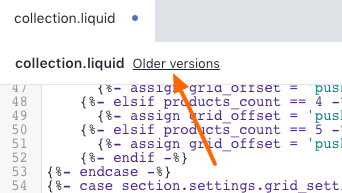# Pagination - Modify how many rows are shown on Collection pages

Pagination on desktop occurs from a value in the liquid code. If you want to have infinite scroll by removing pagination, the value in the file can be changed. You also start pagination earlier if needed by adjusting the same value. Here's how you can modify your pagination value for collection pages.

1

### Verify how many products per row for desktop

In the theme editor verify how many products per row you have configured: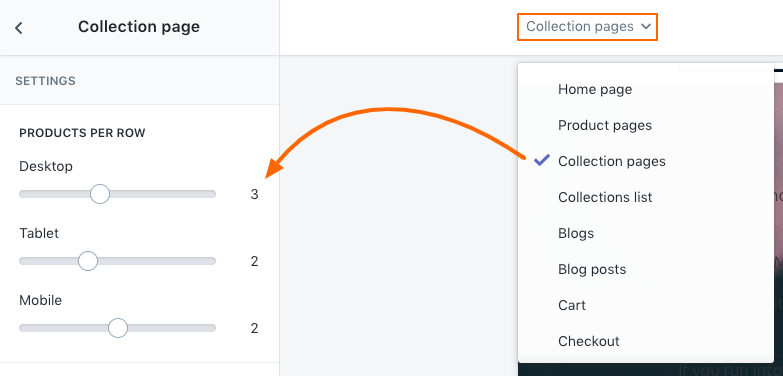2

### Modify the correct liquid line and value

In the Code Editor, modify this file ( Sections folder, collection.liquid):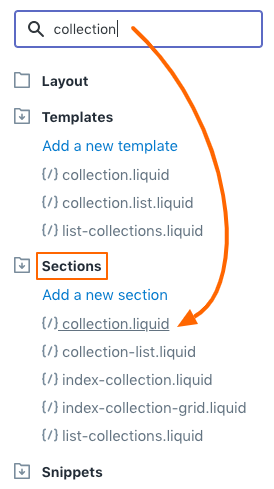Note: For this tutorial, we will work with the setting of 3 for desktop.

Around lines 73 - 83, you can adjust the value for number of items listed based on your grid type: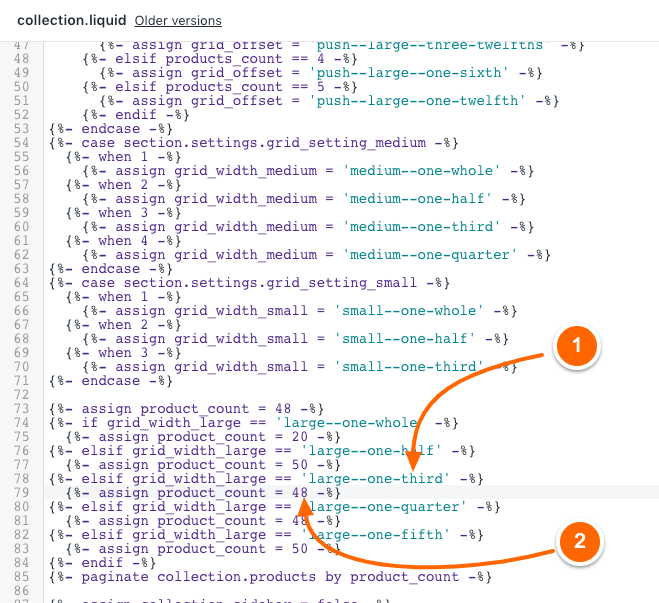(1) Line 78 is referring to 3 items per row (no change to this line, only a reference point).
(2) The value 48 is the number you want to adjust.

Line 79, the value is 48 products per page. If you divide 48 / 3 (number of items per row) = 16
That's how many rows are shown before pagination occurs.

If you want to show an infinite number of products, you can change the value from 48 (Line 79) to something like 9999

Example: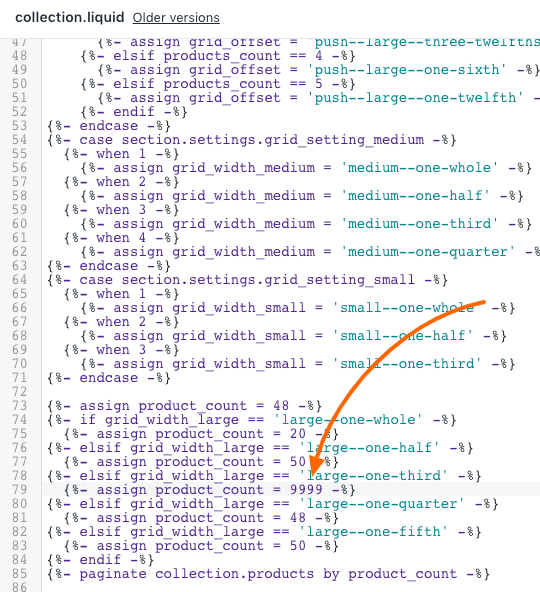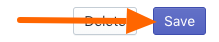Reference:
If your items per row setting is 2, then the correct line to modify is: Line 77
If your items per row setting is 3, then the correct line to modify is: Line 79
If your items per row setting is 4, then the correct line to modify is: Line 81
If your items per row setting is 5, then the correct line to modify is: Line 83
If your items per row setting is 6, then the correct line to modify is: Line 73

*The above reference line numbers can vary based on your version of Pipeline and other modifications.
Use the line above as a reference point to how many are displayed per row. Example:
large--one-half equals 2 per row
large--one-third equals 3 per row
large--one-quarter equals 4 per row.
large--one-fifth equals 5 per row.

#### Notes

• If you wanted pagination to occur earlier, less than 16 rows, you would simply find a value that is divisible by 3 and enter that. Example, short page of only 8 rows would be 8 * 3 = 24. That would only show 8 rows before pagination occurs.
• If you wanted pagination after 30 rows of products, then the calculation is 30 * 3 = 90. Use 90 as the value.
• Using a value like 9999 in most cases would remove pagination (unless you have more than 9999 products).
• If you have 4 products per page, then base your calculation on 4. Example 30 rows is 30 * 4 = 120.

#### Best practice

Make a duplicate/backup of your theme before making any code changes (very important):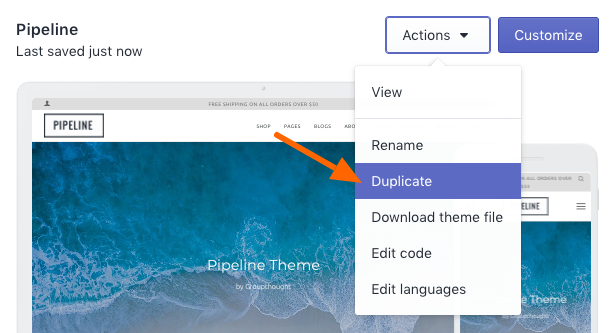#### Undo

There is an undo if you make a mistake with this file. Use the "Older versions" link at the top: